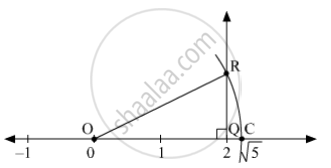SSC (English Medium) Class 8Maharashtra State Board
Share

# Show the Number √5 on the Number Line. - SSC (English Medium) Class 8 - Mathematics

ConceptTo Show Rational Numbers on a Number Line

#### Question

Show the number √5 on the number line.

#### SolutionDraw a number line as shown in the figure. Let the point O represent 0 and point Q represent 2. Draw a perpendicular QR at Q on the number line such that QR = 1 unit. Join OR. Now, ∆OQR is a right angled triangle.
By Pythagoras theorem, we have
OR2 = OQ2 + QR2
= (2)+ (1)2
= 4 + 1
= 5
∴ OR = √5
Taking O as the centre and radius OR = √5, draw an arc cutting the number line at C.
Clearly, OC = OR = √5
Hence, C represents √5 on the number line.

Is there an error in this question or solution?

#### APPEARS IN

Balbharati Solution for Balbharati Class 8 Mathematics (2019 to Current)
Chapter 1: Rational and Irrational numbers
Practice Set 1.4 | Q: 2 | Page no. 6
Solution Show the Number √5 on the Number Line. Concept: To Show Rational Numbers on a Number Line.
S